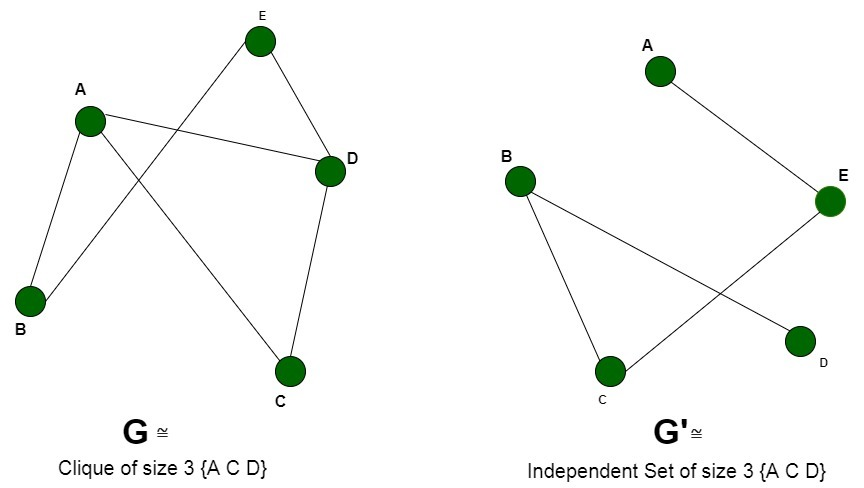Open in App
Not now

# Proof that Independent Set in Graph theory is NP Complete

• Last Updated : 13 Jul, 2022

Prerequisite: NP-Completeness, Independent set.

An Independent Set S of graph G = (V, E) is a set of vertices such that no two vertices in S are adjacent to each other. It consists of non- adjacent vertices.

Problem: Given a graph G(V, E) and an integer k, the problem is to determine if the graph contains an independent set of vertices of size >=k.
Explanation:
An instance of the problem is an input specified to the problem. An instance of the Independent Set problem is a graph G (V, E) and a positive integer k, and the problem is to check whether an independent set of size k exists in G. Since an NP-Complete problem, by definition, is a problem which is both in NP and NP-hard, the proof for the statement that a problem is NP-Complete consists of two parts:

1. The problem itself is in NP class.
2. All other problems in NP class can be polynomial-time reducible to that.
(B is polynomial-time reducible to C is denoted as)

If the 2nd condition is only satisfied then the problem is called NP-Hard.

But it is not possible to reduce every NP problem into another NP problem to show its NP-Completeness all the time. That is why if we want to show a problem is NP-Complete we just show that the problem is in NP and any NP-Complete problem is reducible to that then we are done, i.e. if B is NP-Complete andfor C in NP, then C is NP-Complete.

1. Independent Set is NP
If any problem is in NP, then, given a ‘certificate’, which is a solution to the problem and an instance of the problem (a graph G and a positive integer k, in this case), we will be able to verify (check whether the solution given is correct or not) the certificate in polynomial time.
The certificate is a subset V’ of the vertices, which comprises the vertices belonging to the independent set. We can validate this solution by checking that each pair of vertices belonging to the solution are non-adjacent, by simply verifying that they don’t share an edge with each other. This can be done in polynomial time, that is O(V +E) using the following strategy of graph G(V, E):

```flag=true
For every pair {u, v} in the subset V’:
Check that these two don’t
have an edge between them
If there is an edge,
set flag to false and break
If flag is true:
Solution is correct
Else:
Solution is incorrect
```
2. Independent Set is NP-Hard.
In order to prove that the Independent Set problem is NP-Hard, we will perform a reduction from a known NP-Hard problem to this problem. We will carry out a reduction from which the Clique Problem can be reduced to the Independent Set problem.
Every instance of the clique problem consisting of the graph G (V, E) and an integer k can be converted to the required graph G’ (V’, E’) and k’ of the Independent Set problem. We will construct the graph G’ in the following way:

V’ = V i.e. all the vertices of graph G are a part of the graph G’
E’ = complement of the edges E, i.e. the edges not present in the original graph G.

The graph G’ is the complementary graph of G. The time required to compute the complementary graph G’ requires a traversal over all the vertices and edges. The time complexity of this is O (V+E).
We will now prove that the problem of computing the independent set indeed boils down to the computation of the clique. The reduction can be proved by the following two propositions:

• Let us assume that the graph G contains a clique of size k. The presence of clique implies that there are k vertices in G, where each of the vertices is connected by an edge with the remaining vertices. This further shows that since these edges are contained in G, therefore they can’t be present in G’. As a result, these k vertices are not adjacent to each other in G’ and hence form an Independent Set of size k.
• We assume that the complementary graph G’ has an independent set of vertices of size k’. None of these vertices shares an edge with any other vertices. When we complement the graph to obtain G, these k vertices will share an edge and hence, become adjacent to each other. Therefore, the graph G will have a clique of size k.Thus, we can say that there is an independent set of size k in graph G if there is a clique of size k in G’ (complement graph). Therefore, any instance of the independent set problem can be reduced to an instance of the clique problem. Thus, the independent set is NP-Hard.

Conclusion:
Since the Independent Set problem is both NP and NP-Hard, therefore it is an NP-Complete problem.